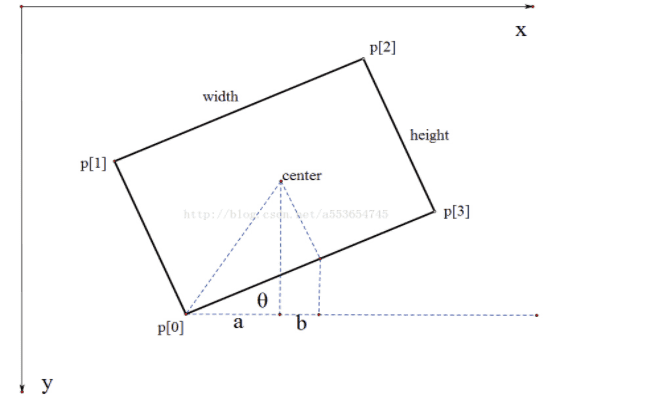API与工具

# 通用识别

### 请求接口

• 云市场接口
• 请求方法: POST
• 请求url: 在云市场搜索`印刷文字识别-通用识别`,在`API接口`中找到`调用地址`

## 输入格式

``{``   "image":    "图片以base64编码的string"，``    "configure":``        "{\"min_size\" : 16,  #图片中文字的最小高度，单位像素``          \"output_prob\" : true}" #是否输出文字框的概率``}``

## 输出格式

``{``    "request_id" : "20170301160849_918cfcae128fc919ad6d6e3b865d2973",     #请求唯一标识，用于错误追踪``    "ret":[``        {``           \"prob\" : 0.95983,  #文字区域概率 ``           "rect":{     #文字区域``                "angle" : 0,   #文字区域角度``                "left" : 50,   #文字区域左上角x坐标``                "top" : 50,   #文字区域左上角y坐标``                "width" : 100,  #文字区域宽度``                "height" : 40     #文字区域高度``            },``            "word":"文字内容"    #文字内容``        },``        {``            \"prob\" : 0.95983,  #文字区域概率``            "rect":{     #文字区域``                "angle" : 10,   #文字区域角度``                "left" : 50,   #文字区域左上角x坐标``                "top" : 50,   #文字区域左上角y坐标``                "width" : 100,  #文字区域宽度``                "height" : 40     #文字区域高度``            },``            "word":"文字内容"    #文字内容``        }``    ],``    "success" : true``}``

### 倾斜框的输出格式：

opencv中旋转矩形框格式为[x_center, y_center, w, h, angle] 注意夹角 angle 定义为，把水平轴逆时针旋转，碰到第一条w边，水平轴和这条边的夹角即为angle, angle的取值范围为(-90,0]。``def makeRectangle(width, height, theta, offset=(0,0)):``    c, s = math.cos(theta), math.sin(theta)``    rectCoords = [(width/2.0, height/2.0), (width/2.0, -height/2.0), (-width/2.0, -height/2.0), (-width/2.0, height/2.0)]``    return [(c*x-s*y+offset, s*x+c*y+offset) for (x,y) in rectCoords]``origin_center_x = left + width/2.0``origin_center_y = top + height/2.0``vertices = makeRectangle(width, height, angle*math.pi/180, (origin_center_x, origin_center_y))``